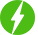MATLAB
• Show all
• Hottest
• Popular
0

## Learn MATLAB from ZERO to HERO

Free \$19.99
-100%

Learn MATLAB from ZERO to HERO, Learn MATLAB without any previous knowledge. MATLAB is one of the fundamental and leading programming languages; therefore, ...

Expand• Total (0)
0